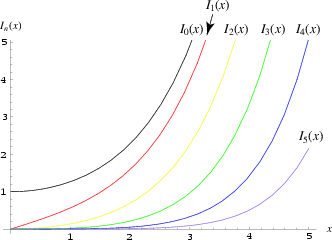•http://facebook.com/
•https://www.google.com/accounts/o8/id
•https://me.yahoo.comCOST (GBP)5.6211.740

# i

viewed 3191 times and licensed 81 times
Modified Bessel function of the first kind of integer order.
Controller: CodeCogsContents## DependentsC++
Excel

## Overview

These function return solutions to the Modified Bessel Function of the first kind.

The differential equation where v is a real constant, is called the modified Bessel's equation, with the solution known as the modified Bessel function, with solutions: and , and where

where is the gamma function.The Bessel function at varying orders A second solution, which is independent of , is known as the modified Bessel function of the kind Maths/Special/Bessel/K/K

## References:

http://mathworld.wolfram.com/ModifiedBesselFunctionoftheFirstKind.html

## I

 doubleI( double x int v )[inline]
Returns modified Bessel function of the first kinds for any integer order (v)

The function is defined as

The range is partitioned into the two intervals [0,8] and (8, infinity). Chebyshev polynomial expansions are employed in each interval.

## Accuracy:

<pre> Relative error: arithmetic domain # trials peak rms DEC 0, 30 3400 1.2e-16 2.3e-17 IEEE 0, 30 30000 1.9e-15 2.1e-16</pre>

## References:

Cephes Math Library Release 2.8: June, 2000

### Parameters

 x value to be transformed. v order of bessel function.

### Authors

Stephen L. Moshier. Copyright 1984, 1987, 2000
Documentation by Will Bateman (August 2005)
##### Source Code

Source code is available when you agree to a GP Licence or buy a Commercial Licence.

Not a member, then Register with CodeCogs. Already a Member, then Login.

## I

 doubleI( double x double v )
Returns modified Bessel function of the first kinds for any order (v)

The function is defined as

The range is partitioned into the two intervals [0,8] and (8, infinity). Chebyshev polynomial expansions are employed in each interval.

## Accuracy:

<pre> Relative error: arithmetic domain # trials peak rms DEC 0, 30 3400 1.2e-16 2.3e-17 IEEE 0, 30 30000 1.9e-15 2.1e-16</pre>

## Example:

#include <codecogs/maths/special/bessel/i/i.h>
#include <stdio.h>

int main()
{
using namespace Maths::Special::Bessel::I;
printf("\n  x      v=0      v=1      v=2      v=3      v=4      v=5");
for(double x=0; x<6; x++)
{
printf("\nx=%.1lf",x);
for(int v=0;v<=5;v++)
printf(" %8.6lf", I(x,v));
}
return 0;
}

## Output:

x      v=0      v=1      v=2      v=3      v=4      v=5
x=0.0 1.000000 0.000000 0.000000 0.000000 0.000000 0.000000
x=1.0 1.266066 0.565159 0.135748 0.022168 0.002737 0.000271
x=2.0 2.279585 1.590637 0.688948 0.212740 0.050729 0.009826
x=3.0 4.880793 3.953370 2.245212 0.959754 0.325705 0.091206
x=4.0 11.301922 9.759465 6.422189 3.337276 1.416276 0.504724
x=5.0 27.239872 24.335642 17.505615 10.331150 5.108235 2.157975

## References:

Cephes Math Library Release 2.8: June, 2000

### Parameters

 x input argument. v order of bessel function.

### Authors

Stephen L. Moshier. Copyright 1984, 1987, 2000
Documentation by Will Bateman (August 2005)
##### Source Code

Source code is available when you agree to a GP Licence or buy a Commercial Licence.

Not a member, then Register with CodeCogs. Already a Member, then Login.

Last Modified: 18 Oct 07 @ 17:07     Page Rendered: 2022-03-14 15:00:34